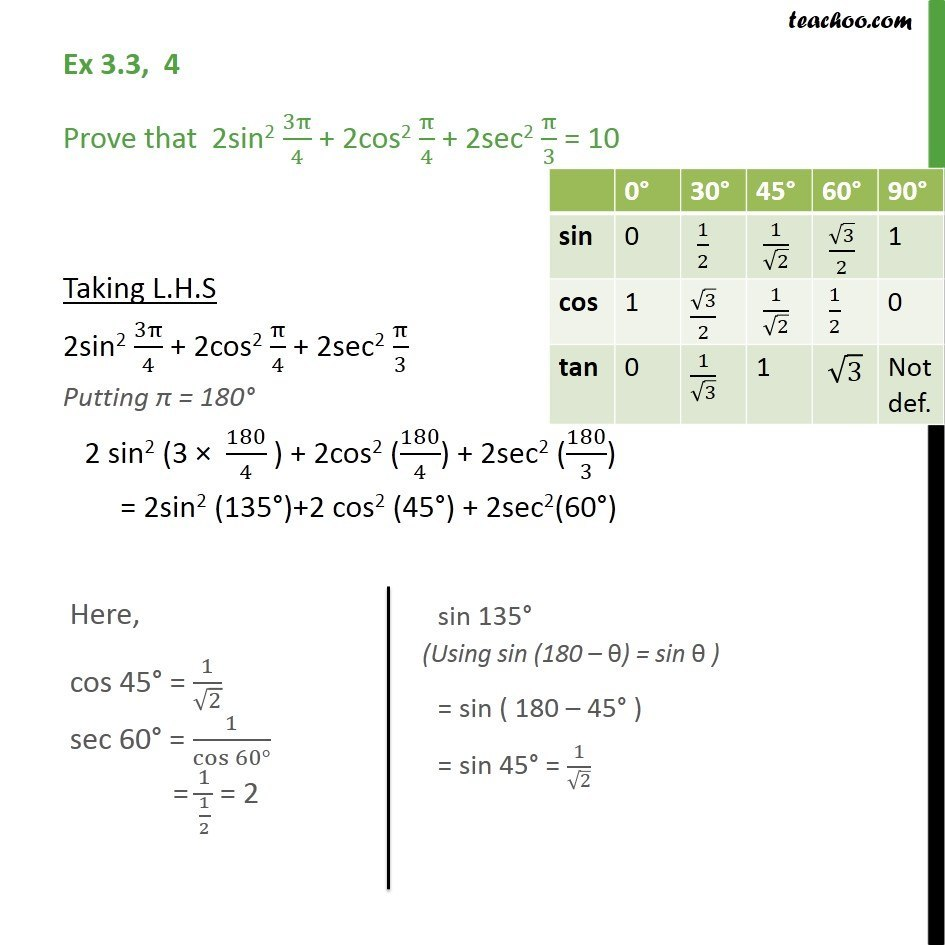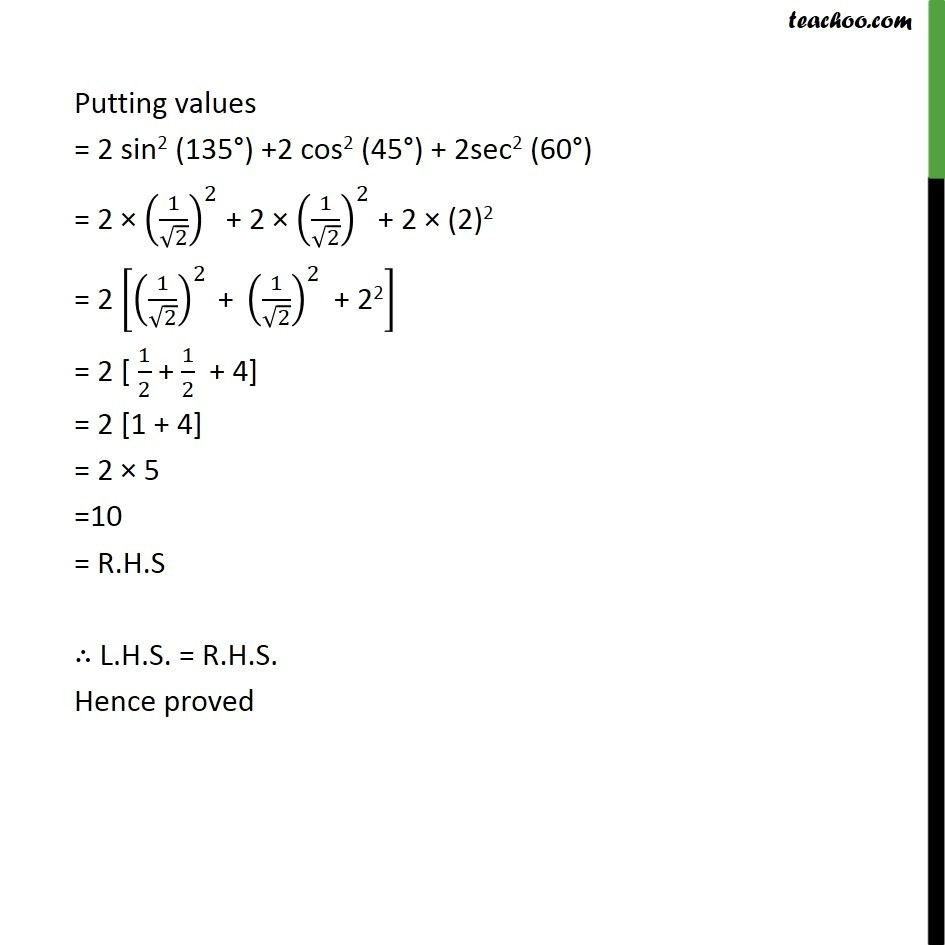Learn All Concepts of Chapter 2 Class 11 Relations and Function - FREE. Check - Trigonometry Class 11 - All Concepts1. Chapter 3 Class 11 Trigonometric Functions
2. Serial order wise
3. Ex 3.3

Transcript

Ex 3.3, 4 Prove that 2sin2 3 /4 + 2cos2 /4 + 2sec2 /3 = 10 Taking L.H.S 2sin2 3 /4 + 2cos2 /4 + 2sec2 /3 Putting = 180 2 sin2 (3 180/4 ) + 2cos2 (180/4) + 2sec2 (180/3) = 2sin2 (135 )+2 cos2 (45 ) + 2sec2(60 ) Putting values = 2 sin2 (135 ) +2 cos2 (45 ) + 2sec2 (60 ) = 2 (1/ 2)^2 + 2 (1/ 2)^2 + 2 (2)2 = 2 [(1/ 2)^2 " + " (1/ 2)^2 " + 22" ] = 2 [ 1/2 + 1/2 + 4] = 2 [1 + 4] = 2 5 =10 = R.H.S L.H.S. = R.H.S. Hence proved

Ex 3.3